Get instant live expert help with Excel or Google Sheets“My Excelchat expert helped me in less than 20 minutes, saving me what would have been 5 hours of work!”

#### Post your problem and you’ll get expert help in seconds.

Your message must be at least 40 characters
Our professional experts are available now. Your privacy is guaranteed.

# How To Make A Correlation Matrix In Excel

A matrix is a set of numbers arranged in rows and columns in a specific format. We can construct a correlation matrix to measure dependency or relationships between two or more variables. In this tutorial, we will learn how to create a correlation matrix for two variables as well as multiple variable scenarios.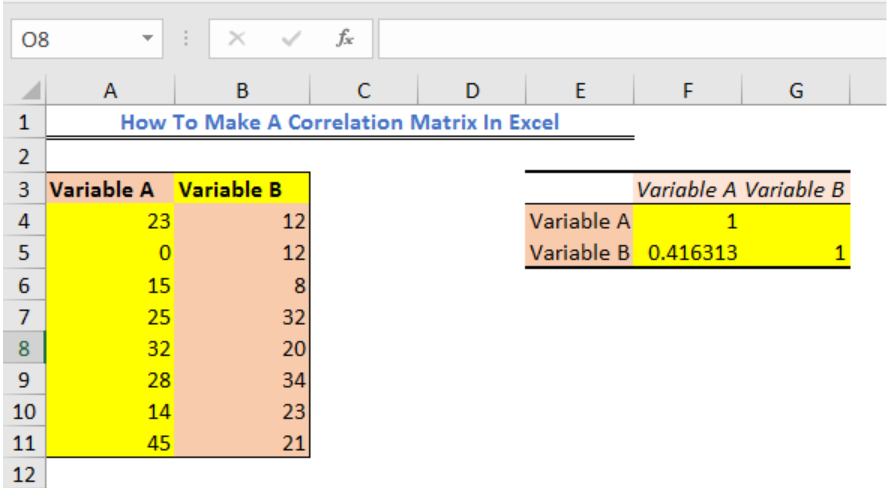Figure 1 – How to create a correlation matrix in excel

## Excel Correlation Matrix

The correlation matrix will show how one variable is dependent on the other variable. The resulting coefficients can be positive, negative, or zero. i.e., -1, +1 or 0. This means;

• “+1” – Positive correlation coefficient resulting when both variables are moving in the same direction
• “-1” – Negative Correlation coefficient resulting when the variables are moving in oppositive directions
• “0” or blank cell – Zero Correlation Coefficient when variables are not dependent on each other.

## Correlation Matrix using Data Analysis

We need to enable the Analysis ToolPak to do the Correlation Matrix. We will go to File and select Options.Figure 2 – Making Excel Correlation Matrix.

• In the Add-Ins window, we will choose Excel Add-Ins from the Manage field and click Go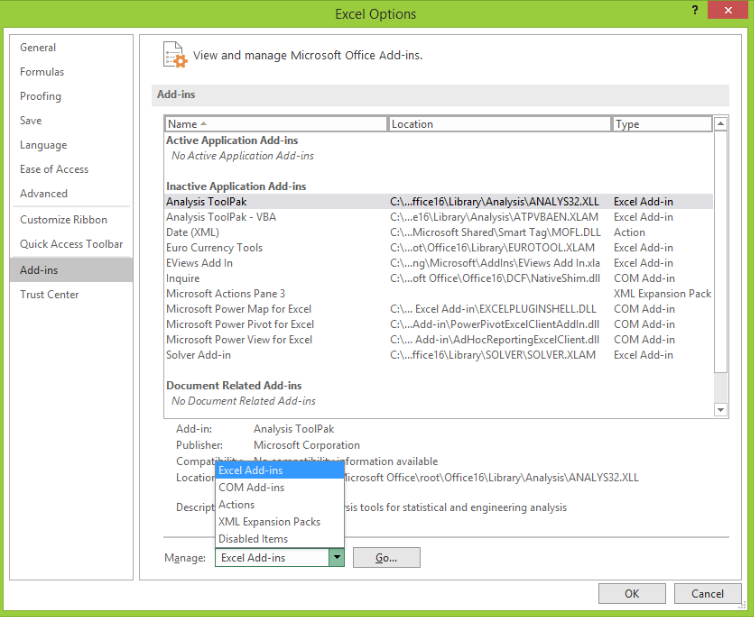Figure 3 – Activating the Analysis ToolPak for creating a correlation matrix in excel

• Next, we will mark the option for Analysis ToolPak and click OK.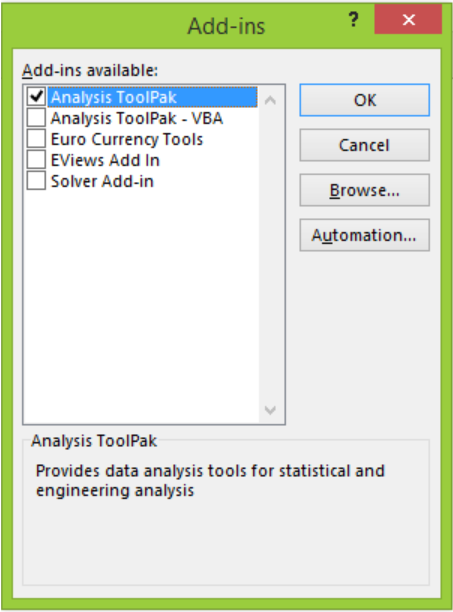Figure 4 – Activating Analysis ToolPak for correlation in excel 2016

## Create Correlation Matrix using two variables

• We will prepare our data table.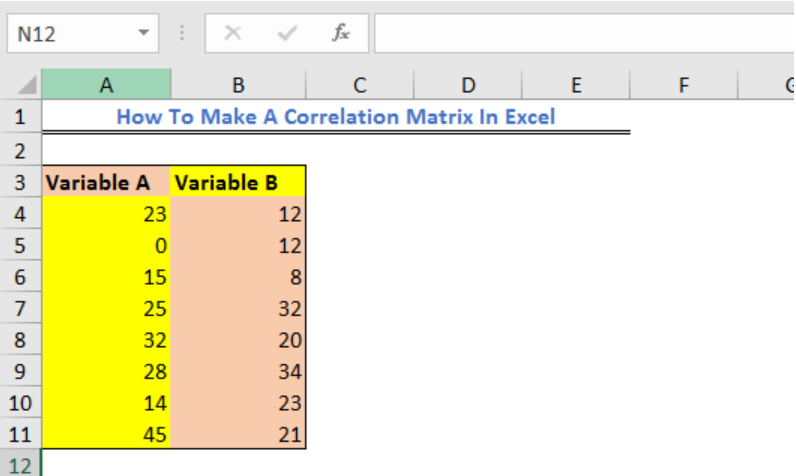Figure 5 – How to make a correlation matrix in excel

• We will go to the Data Tab and select Data Analysis.

v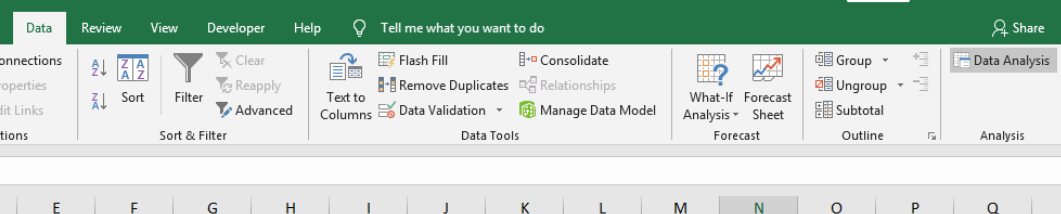Figure 6 – Create Correlation Matrix in Excel

• In the Data Analysis pop-up window, we will select Correlation and select OK.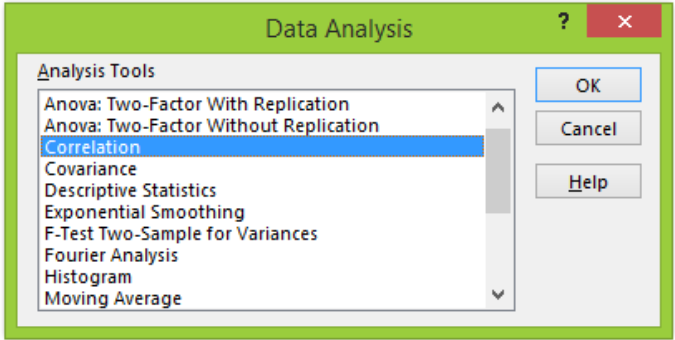Figure 7 – Excel Correlation Matrix

• In the Correlation dialog box,
• we will select our data range

• We will mark label in first row

• Next, we will select the Output range and finally select OK.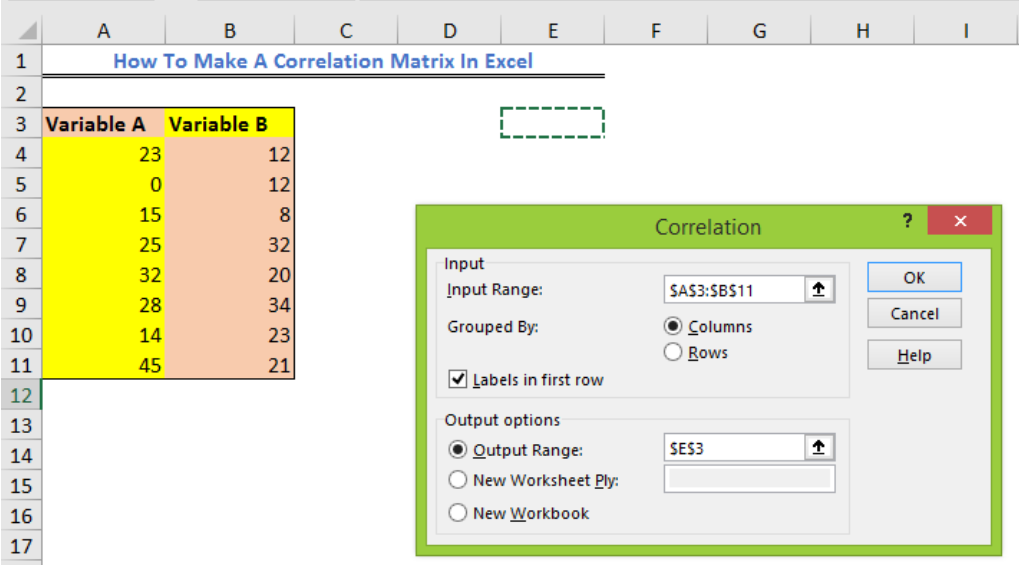Figure 8 – How to do a correlation matrix in excel

• We will have this result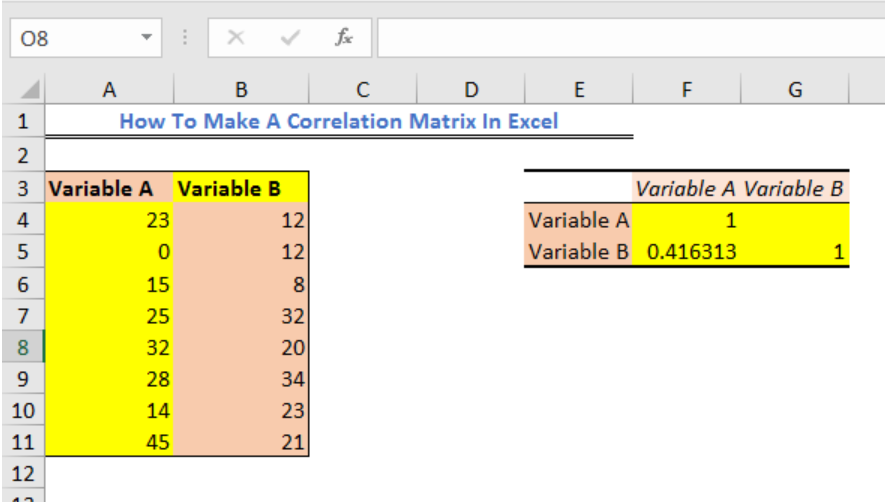Figure 9 – How to Calculate a correlation matrix in excel

## Correlation Matrix using multiple variables

• We will prepare our data table.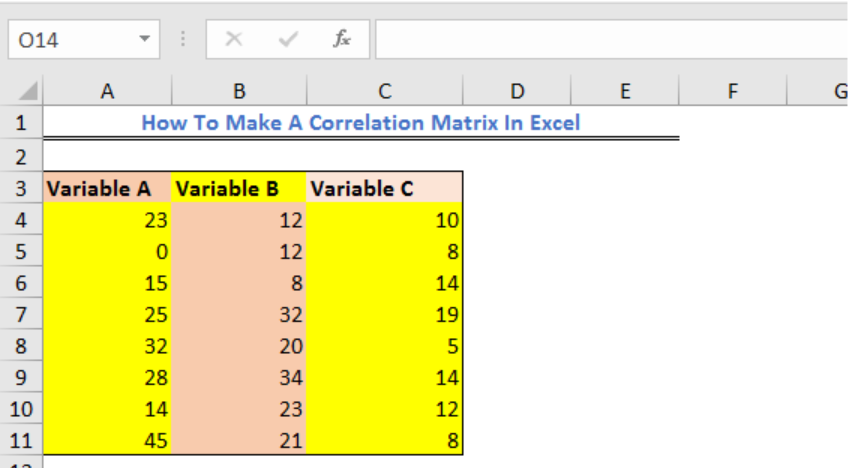Figure 10 – How to do a correlation test in excel

• We will go to the Data Tab and select Data Analysis.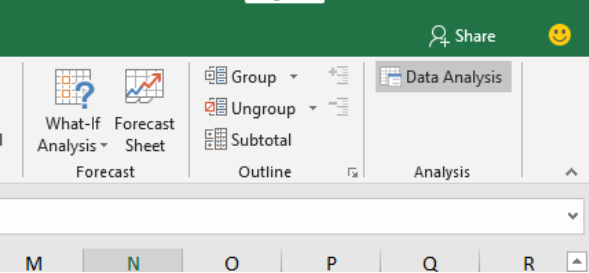Figure 11 – Enable Data analysis for excel correlation matrix

• In the Data Analysis pop-up window, we will select Correlation and select OK.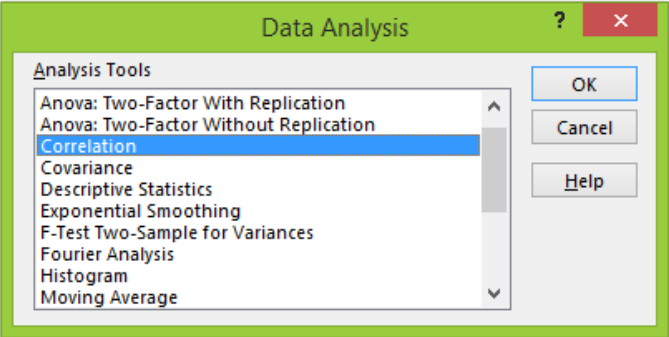Figure 12 – Enable Correlation matrix in Excel

• In the Correlation dialog box,
• We will select our data range

• We will mark label in first row

•
• Next, we will select the Output range and finally select OK.

Figure 13 – How to calculate the correlation matrix in excel

• We will have this result

Figure 14 – How to make a correlation matrix in excel

## Instant Connection to an Excel Expert

Most of the time, the problem you will need to solve will be more complex than a simple application of a formula or function. If you want to save hours of research and frustration, try our Excelchat service! Our Excel Experts are available 24/7 to answer any Excel question you may have. We guarantee a connection within 30 seconds and a customized solution within 20 minutes.